# Class 10 Maths NCERT Solutions for Similar triangles EXERCISE 6.5

In this page we have Class 10 Maths NCERT Solutions for Similar triangles for EXERCISE 6.5 on pages 150 and 151. Hope you like them and do not forget to like , social_share and comment at the end of the page.
Formula Used in this exercise
Pythagoras Theorem for right angle triangle ABC , right angle at B
$AC^2 = AB^2 + BC^2$

## Triangles EXERCISE 6.5

Question 1
Sides of triangles are given below. Determine which of them are right triangles? In case of a right triangle, write the length of its hypotenuse.
(i) 7 cm, 24 cm, 25 cm
(ii) 3 cm, 8 cm, 6 cm
(iii) 50 cm, 80 cm, 100 cm
(iv) 13 cm, 12 cm, 5 cm
(i) Given that the sides of the triangle are 7 cm, 24 cm, and 25 cm.
Squaring the lengths of these sides, we will get 49, 576, and 625.
$49 + 576 = 625$
$(7)^2 + (24)^2= (25)^2$
The sides of the given triangle are satisfying Pythagoras triplet.Hence, it is an right angled triangle.
Hypotenuse is the longest side in the triangle
Length of Hypotenuse = 25 cm
(ii) Given that the sides of the triangle are 3 cm, 8 cm, and 6 cm.
Squaring the lengths of these sides, we get 9, 64, and 36.
but $9 + 36 \ne 64$
Or, $3^2 + 6^2 \ne 8^2$
So, the sum of the squares of the lengths of two sides is not equal to the square of the length of the third side.
Hence, the given triangle is not satisfying Pythagoras theorem and it is not a right angle triangle
(iii)Given that sides are 50 cm, 80 cm, and 100 cm.
Squaring the lengths of these sides, we get 2500, 6400, and 10000.
But, $2500 + 6400 \ne 10000$
Or, $50^2+ 80^2 \ne 100^2$
Clearly, the sum of the squares of the lengths of two sides is not equal to the square of the length of the third side.
Therefore, the given triangle is not satisfying Pythagoras theorem.
So, it is not a right triangle.
(iv) Given that sides are 13 cm, 12 cm, and 5 cm.
Squaring the lengths of these sides, we will get 169, 144, and 25.
Now, $144 +25 = 169$
Or, $12^2+ 5^2= 13^2$
The sides of the given triangle are satisfying Pythagoras theorem.
Therefore, it is a right triangle.
Length of the hypotenuse of this triangle is 13 cm.

Question 2
PQR is a triangle right angled at P and M is a point on QR such that PM ⊥ QR. Show that $PM^2= QM \times MR$.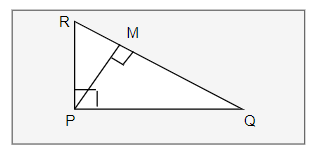In Right Angle ΔPQR, we have by Pythagoras theorem
$QR^2 = PQ^2 + PR^2$ -(i)
In Right Angle ΔPQM, we have by Pythagoras theorem
$PQ^2= PM^2+ QM^2$
Or, $PM^2= PQ^2- QM^2$ ...(ii)
In Right angle ΔPMR, we have by Pythagoras theorem
$PR^2= PM^2+ MR^2$
Or, $PM^2= PR^2- MR^2$ ...(iii)
$2PM^2=(PQ^2+ PM^2) - (QM^2+ MR^2)$
$= QR^2- QM^2- MR^2$
Substituting the value of QA from (i)
$2PM^2 = (QM+ MR)^2- QM^2- MR^2$
$2PM^2= 2QM \times MR$
Therefore
$PM^2= QM \times MR$
Question 3
ABD is a triangle right angled at Aand AC ⊥ BD. Show that
(i) $AB^2= BC \times BD$
(ii) $AC^2= BC \times DC$
(iii) $AD^2= BD \times CD$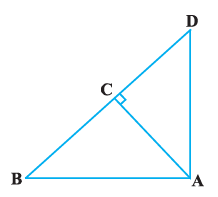(i) In ΔADB and ΔCAB, we have
∠DAB = ∠ACB (Each equals to 90°)
∠ABD = ∠CBA (Common angle)
By AA similarity criterion
Now sides will be proportional
$\frac {AB}{CB} = \frac {BD}{AB}$
$AB^2= CB \times BD$
(ii)
In ΔCBA,
∠CBA = 180° - 90° -∠CAB
∠CBA = 90° -∠CAB
∠CDA = 180° - 90° - (90° -∠CAB)
∠CDA =∠CAB
In ΔCBA and ΔCAD, we have
∠CAB = ∠CDA
∠ACB = ∠DCA (Each equals to 90°)
By AAA similarity criterion
Now sides will be proportional
$\frac {AC}{DC} = \frac {BC}{AC}$AC^2= DC \times BC$(iii) In ΔDCA and ΔDAB, we have ∠DCA = ∠DAB (Each equals to 90°) ∠CDA = ∠ADB (common angle) By AA similarity criterion ΔDCA ~ ΔDAB Now sides will be proportional$\frac {DC}{DA} = \frac {DA}{DA} AD^2= BD \times CD$Question 4 ABC is an isosceles triangle right angled at C. Prove that$AB^2= 2AC^2$. Answer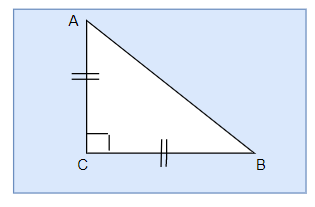Given that ΔABC is an isosceles triangle right angled at C. By using Pythagoras theorem$AB^2= AC^2+ BC^2$Now AC = BC Therefore$AB^2 = AC^2+AC^2AB^2= 2AC^2$Question 5 ABC is an isosceles triangle with AC = BC. If$AB^2= 2AC^2$, prove that ABC is a right triangle. AnswerIn ΔACB, AC = BC (Given)$AB^2= 2AC^2$(Given)$AB^2= AC^2+ AC^2AB^2= AC^2+ BC^2$[Since, AC = BC] Hence, By Pythagoras theorem ΔABC is right angle triangle. Question 6 ABC is an equilateral triangle of side 2a. Find each of its altitudes. Answer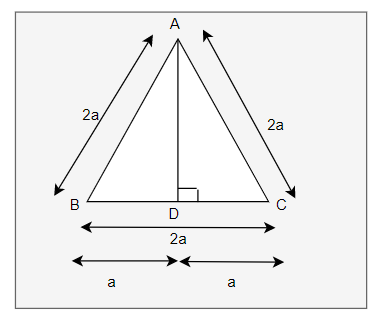Draw, AD⊥BC In ΔADB and ΔADC, we have AB = AC [Given] AD = AD [Given] ∠ADB = ∠ADC [equal to 90°] By RHS congruence. Therefore, ΔADB≅ΔADC By CPCT Hence, BD = DC In right angled ΔADB,$AB^2= AD^2+ BD^2(2a)^2= AD^2 +a^2AD^2= 4a^2-a^2AD^2= 3a^2$or$AD= \sqrt {3} a$Question 7 Prove that the sum of the squares of the sides of rhombus is equal to the sum of the squares of its diagonals. Answer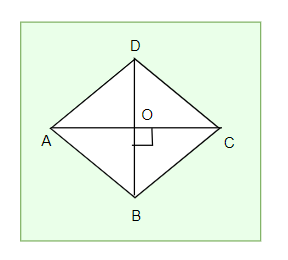Since, the diagonals of a rhombus bisect each other at right angles. Therefore, AO = CO and BO = DO In ΔAOB, ∠AOB =90° By Pythagoras theorem$AB^2= AO^2+ BO^2$...(i) Similarly,$AD^2= AO^2+ DO^2$...(ii)$DC^2= DO^2+ CO^2$...(iii)$BC^2= CO^2+ BO^2$...(iv) Adding equations(i) + (ii)+ (iii)+ (iv) we get,$AB^2+ AD^2+DC^2+BC^2 = 2(AO^2+ BO^2+ DO^2+ CO^2)= 4AO^2+ 4BO^2$Since, AO = CO and BO =DO$AB^2+ AD^2+DC^2+BC^2 = (2AO)^2+ (2BO)^2= AC^2+ BD^2$Question 8 O is a point in the interior of a triangle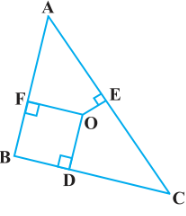ABC, OD ⊥ BC, OE⊥AC and OF ⊥ AB. Show that (i)$OA^2+ OB^2+ OC^2 - OD^2 - OE^2 - OF^2= AF^2+ BD^2+ CE^2$(ii)$AF^2+ BD^2+ CE^2= AE^2+ CD^2+ BF^2$. Answer Join OA, OB and OC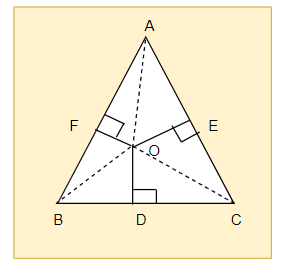(i) In right angle triangle ΔAOF, ByPythagoras theorem$OA^2= OF^2+AF^2$Similarly, in right angle triangle ΔBOD$OB^2= OD^2+ BD^2$Similarly,in right angle triangle ΔCOE$OC^2= OE^2+ EC^2$Adding these equations,$OA^2+ OB^2+ OC^2= OF^2+ AF^2+ OD^2+ BD^2+ OE^2+ EC^2OA^2+ OB^2+ OC^2 - OD^2 - OE^2 - OF^2= AF^2+ BD^2+ CE^2$(ii)$AF^2+ BD^2+ EC^2= (OA^2- OE^2)+ (OC^2- OD^2)+ (OB^2- OF^2)$∴$AF^2+ BD^2+ CE^2= AE^2+ CD^2+ BF^2$. Question 9 A ladder 10 m long reaches a window 8 m above the ground. Find the distance of the foot of the ladder from base of the wall. Answer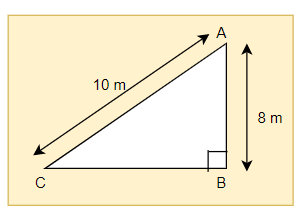Let AB be the wall and AC be the ladder, Now in Right angle triangle ABC,by Pythagoras theorem,we have$AC^2=AB^2+ BC^210^2= 8^2+ BC^2BC^2= 36$BC= 6m Therefore, the distance of the foot of the ladder from the base of the wall is6 m. Question 10 A guy wire attached to a vertical pole of height 18 m is 24 m long and has a stake attached to the other end. How far from the base of the pole should the stake be driven so that the wire will be taut ? Answer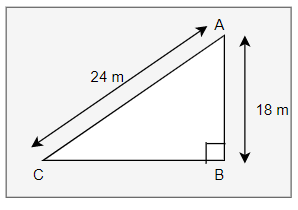Let AB be the pole and AC be the wire. Now in Right angle triangle ABC,by Pythagoras theorem,we have$AC^2=AB^2+ BC^224^2= 18^2+ BC^2BC^2= 252$BC= 6√7m Question 11. An aeroplane leaves an airport and flies due north at a speed of 1,000 km per hour. At the same time, another aeroplane leaves the same airport and flies due west at a speed of 1,200 km per hour. How far apart will be the two planes after$1 \frac {1}{2}$hours? Answer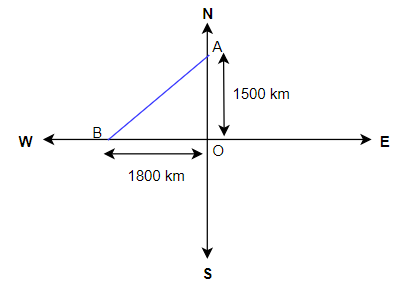Speed of first aeroplane = 1000 km/hr Now Distance = Speed * time So, Distance covered by first aeroplane due north in$1 \frac {1}{2}$hours (OA) = 100 × 3/2 km = 1500 km Speed of second aeroplane = 1200 km/hr so, Distance covered by second aeroplane due west in$1 \frac {1}{2}$hours (OB) = 1200 × 3/2 km = 1800 km In right angle ΔAOB, we have$AB^2=AO^2+ OB^2AB^2=(1500)^2+ (1800)^2$AB = 300√61km Therefore , the distance between two aeroplanes will be 300√61km. Question 12 Two poles of heights 6 m and 11 m stand on a plane ground. If the distance between the feet of the poles is 12 m, find the distance between their tops. Answer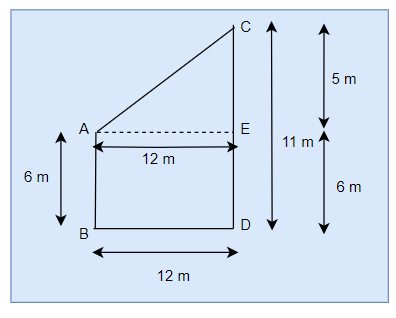Let CD and AB be the poles of height 11 m and 6 m. Therefore, CP = 11 - 6 = 5 m Now BD=AE= 12m Applying Pythagoras theorem for ΔAEC, we get$AC^2=AE^2+ CE^2AC^2= 144+25 = 169$AC = 13m Therefore, the distance between their tops is 13 m. Question 13 D and E are points on the sides CA and CB respectively of a triangle ABC right angled at C. Prove that$AE^2+ BD^2= AB^2+ DE^2$. Answer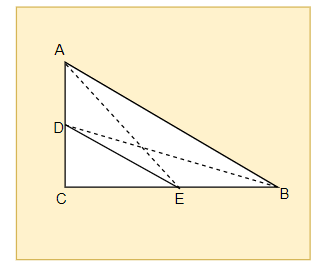Applying Pythagoras theorem in right angle ΔACE, we get$AC^2+CE^2= AE^2$....(i) Applying Pythagoras theorem in right angle ΔBCD, we get$BC^2+CD^2= BD^2$....(ii) Using equations(i)and(ii), we get$AC^2+CE^2+ BC^2+CD^2= AE^2+ BD^2$...(iii) Applying Pythagoras theorem in right angle ΔCDE, we get$DE^2=CD^2+ CE^2$Applying Pythagoras theorem in right angle ΔABC, we get$AB^2=AC^2+ CB^2$Putting these values in equation(iii), we get$AE^2+ BD^2 =DE^2+ AB^2$Question 14 The perpendicular from A on side BC of aΔ ABC intersects BC at D such that DB = 3CD Prove that$2AB^2= 2AC^2+ BC^2$.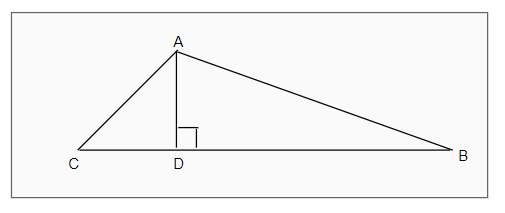Answer Given that in ΔABC, we have AD ⊥BC and BD = 3CD In right angle triangles ADB and ADC, we have by Pythagoras theorem$AB^2=AD^2+ BD^2$...(i)$AC^2=AD^2+ DC^2$...(ii) Subtracting equation(ii)from equation(i), we get$AB^2- AC^2= BD^2- DC^2$Now BC = BD + CD= 4 CD or CD = BC/4 Also BD = 3 CD = 3 BC/4 Substituting these value of CD and BD, we get$AB^2 - AC^2 = \frac {BC^2}{2}2AB^2= 2AC^2+ BC^2$. Question 15 In an equilateral triangle ABC, D is a point on side BC such that$BD = \frac {1}{3} BC$. Prove that$9AD^2= 7AB^2$. Answer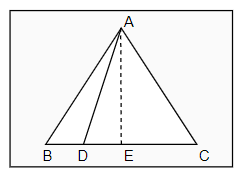Let the side of the equilateral triangle bea, and AE be the altitude of ΔABC. Then BE = EC = BC/2 =a/2 And in right angle ABE$AB^2 = AE^2 + BE^2 AE^2 = AB^2 - BE^2 = a^2 - \frac {a^2}{4}AE = \frac { a \sqrt {3}}{2}$Given that, BD = 1/3BC ∴ BD =a/3 DE = BE - BD =a/2 -a/3 =a/6 Applying Pythagoras theorem in ΔADE, we get AD^2= AE^2+ DE^2$AD^2 = (\frac {a \sqrt {3} }{2})^2 + (\frac {a}{6})^2AD^2 = \frac {7}{9} a^2 9 AD^2 = 7 a^2 = 7 AB^2$or$9AD^2= 7AB^2$. Question 16 In an equilateral triangle, prove that three times the square of one side is equal to four times the square of one of its altitudes. Answer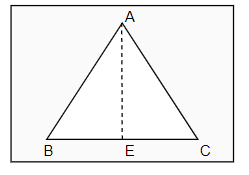Let the side of the equilateral triangle be a, and AE be the altitude of ΔABC. ∴ BE = EC = BC/2 =a/2 And in right angle ABE ,Applying Pythagoras theorem$AB^2 = AE^2 + BE^2 AE^2 = AB^2 - BE^2 = a^2 - \frac {a^2}{4}AE = \frac { a \sqrt {3}}{2}4AE^2 = 3 a^2 $4 × (Square of altitude) = 3 × (Square of one side) Question 17 Tick the correct answer and justify: In ΔABC, AB = 6√3cm, AC = 12 cm and BC = 6 cm. The angle B is: (A) 120° (B) 60° (C) 90° (D) 45° Answer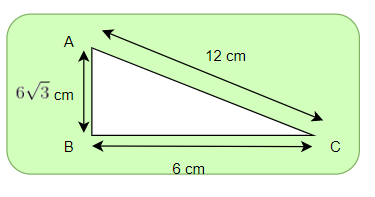Given that, AB =6√3cm,AC = 12 cm, and BC = 6 cm Squaring all the sides and we can observe that$AB^2= 108AC^2= 144$And,$BC^2= 36AB^2+ BC^2= AC^2$Sotriangle, ΔABC, is satisfying Pythagoras theorem. Hencethe triangle is a right triangle, right-angled at B. ∴ ∠B = 90° the correct option is (C). ## Summary 1. NCERT Solutions for Class 10 Maths: Chapter 6 triangles Exercise 6.5 has been prepared by Expert with utmost care. If you find any mistake.Please do provide feedback on mail.You can download this as pdf 2. This chapter 6 has total 5 Exercise 6.1 ,6.2,6.3 ,6.4 and 6.5. This is the Fifth exercise in the chapter.You can explore previous exercise of this chapter by clicking the link below link to this page by copying the following text Also ReadGo back to Class 10 Main Page using below links ### Practice Question Question 1 What is$1 - \sqrt {3}\$ ?
A) Non terminating repeating
B) Non terminating non repeating
C) Terminating
D) None of the above
Question 2 The volume of the largest right circular cone that can be cut out from a cube of edge 4.2 cm is?
A) 19.4 cm3
B) 12 cm3
C) 78.6 cm3
D) 58.2 cm3
Question 3 The sum of the first three terms of an AP is 33. If the product of the first and the third term exceeds the second term by 29, the AP is ?
A) 2 ,21,11
B) 1,10,19
C) -1 ,8,17
D) 2 ,11,20